## Saturday, November 28, 2020

### The Uniform-Distribution-on-the-Sphere's Nemesis: Isothermal CoordinatesInspired by the last post where the conformal version of the polar grid is $(x,y) = (e^u \cos v, e^u \sin v)$ i.e. the radius is now exponentiated (namely what looks like a grid of squares in the domain gets mapped to what looks like squares in the range: the concentric circles bunch up much more densely close to the origin, to correspond to radial lines converging at the origin), I set out to do it on the sphere as well (that conformal polar grid is simply the image of the complex plane under the complex exponential $e^z$). This is in some sense the polar (hah) opposite of something that would produce a uniform distribution of points on a sphere, because this map would assign each square an equal chance (so of course, the closer you get to the poles, the squares are smaller and more of them encompass the same space in the image, so they'll be much more densely packed).

The quickest way to see this is to note the stereographic projection is a conformal map, and has the formula
$(x,y) \mapsto \left(\frac{2x}{x^2+y^2+1}, \frac{2y}{x^2+y^2+1}, \frac{x^2+y^2-1}{x^2+y^2+1}\right)$
and with a substitution of the complex exponential, you get $(u,v) \mapsto \left(\frac{2e^u \cos v}{e^{2u}+1}, \frac{2e^u \sin v}{e^{2u}+1}, \frac{e^{2u}-1}{e^{2u}+1}\right) = \left(\frac{2}{e^u+e^{-u}}\cos v, \frac{2}{e^u+e^{-u}}\sin v, \frac{e^u-e^{-u}}{e^u+e^{-u}}\right)$
I rewrote that in that form for fans of hyperbolic functions, which gives the map
$(u,v) \mapsto(\operatorname{sech} u \cos v, \operatorname{sech} u \sin v, \tanh u)$ (hyperbolic secant seems to be one of the least used ones; $\LaTeX$ doesn't recognize it!). But the upshot is, this formula looks very similar to the usual geographic coordinate system (just swapping out some transcendental functions).

Another way of looking at this is via manipulation of the metric coefficients directly, which raises some interesting points on the nature of the Laplace equation. If we consider the standard geographic parametrization$(x,y,z) = (\sin\varphi\cos\theta, \sin\varphi\sin\theta, \cos\varphi)$ with Euclidean metric $g = ds^2 = d\varphi^2 + \sin^2 \varphi\; d\theta^2$. To say that the parametrization is conformal (grid squares to grid squares) is to say that there are some parameters $u$ and $v$ and a function $\sigma$ such that $g = \sigma^2(du^2 + dv^2)$ (forcing the matrix to not only be diagonal, but a multiple of the identity). If we set things up so that $du = -\csc\varphi d\varphi$ and $dv = d\theta$, then $\sin^2\varphi (du^2 + dv^2) = d\varphi^2 + \sin^2\varphi\; d\theta^2)$. Consulting trusty integral tables, Wolfram Alpha'ing it, or whatever, we find that this differential equation gives $u = \ln(\csc \varphi + \cot \varphi) + C$. One can make it this derivation a little less "out of the blue" situation in a very interesting derivation of Laplace's equation using certain complex analysis hackery (George Springer's Introduction to Riemann Surfaces, section 1-3). We'll derive this another day, but the summary is, we end up deriving that $u$ has to satisfy the spherical Laplace equation $\frac{1}{\sin\varphi} \frac{\partial}{\partial \varphi}\left(\sin \varphi \frac{\partial u}{\partial \varphi}\right) + \frac{1}{\sin \varphi} \frac{\partial^2 u}{\partial \theta^2} = 0,$ or really, it only has to satisfy this on an open subset of the sphere, in this case, a sphere with two points deleted. This fact often gets glossed over by people talking about geometry; we know that on a sphere, the only harmonic functions that are actually defined on the sphere everywhere are constant functions—so we can only find "interesting" harmonic functions on a sphere if they blow up at certain points. Using the very reasonable assumption that such a function $u$ should be independent of $\theta$, we derive $\frac{d}{d \varphi} \left(\sin\varphi \frac{d u}{d \varphi}\right) = 0$, making $\sin\varphi \frac{d u}{d \varphi} = C_1$ a constant, and $\frac{du}{d\varphi} = C_1 \csc \varphi$ to finally get $u = C_1 \ln(\csc \varphi + \cot \varphi) + C_2$, as before (just with two degrees of freedom, namely $C_1$ controls what the initial spacing of the grid lines, and $C_2$ controls what the value is at the equator). This function blows up at the poles, a fact which manifests itself visually by the grid curves $u =$ constant become more closely spaced together at the poles (and become infinitely dense there, meaning, this is only a valid coordinate system in the sphere outside the poles). We just set $C_1 = 1$ and $C_2 = 0$, which assigns the equator to $u=0$.

Finally, we have to do some algebra, though, to get to where we want: trying to find the inverse of $u = \ln(\csc \varphi + \cot \varphi)$. This says $e^u = \csc\varphi+\cot\varphi = \frac{1+\cos\varphi}{\sin\varphi}$. This is just $\frac{1+z}{r}$ where $r$ is the cylindrical coordinate $\sqrt{x^2+y^2}$. But if we're on the sphere, $z^2 + r^2 =1$, so this gives us $e^{2u} = \frac{(1+z)^2}{1-z^2} = \frac{1+z}{1-z}.$ Rearranging we get $e^{2u}-e^{2u}z = 1+z$, or $z(1+e^{2u}) = e^{2u}-1$. This gives $z=\frac{e^{2u}-1}{e^{2u}+1} = \frac{e^{u}-e^{-u}}{e^{u}+e^{-u}} = \tanh u.$. To get the $x$ and $y$, we note that $\sin\varphi = r = e^{-u}(1+z) = e^{-u}(1+\tanh u)$. Finally,
$1+ \tanh u = \frac{\cosh u + \sinh u}{\cosh u} = \frac{e^u}{\cosh u},$ which, multiplying by the $e^{-u}$ gives $\frac{1}{\cosh u}$ or $\operatorname{sech} u$.

The coordinates we derived are called isothermal coordinates, and knowing what a big fan I am of stat mech, I'd like to fully understand the origin of the term someday. Finally, one might ask if there are coordinate systems (conformal or otherwise) that will cover up all the sphere without weird coordinate singularities like that. The answer is no, and the short explanation of why is because a smooth vector field on the sphere must vanish somewhere (the hairy ball theorem), and since coordinates always set up vector fields (take the vector in the direction of a coordinate curve) and aren't supposed to collapse at good points. This is a long topic for another day (we'll show some pretty cool coordinate systems on the sphere, though!)

## Saturday, October 31, 2020

### Happy Halloween from Nested Tori!

Spooky greetings. A quick post with more complex analysis for you. Though maybe not as visually interesting as fractals, but getting back to some basics and/or nuance regarding complex functions. For one thing, I just never had a chance to really fit in a good study of Riemann surfaces (interest in them was partially stirred on by all that recent cubic hackery). Also, a lot of stuff involving branch cuts and branch points are something quickly gone over in standard complex analysis and never really explored in depth, other than to say "we take the principal branch of the logarithm/root/power" function to be... and you don't get into the nuance with the details of differences between different branches of functions. This example here is exploring the mapping $z \mapsto \sqrt{z^2 + 1}$. This is already interesting from the Riemann surface point of view, because neither the function nor its inverse is definable in its most interesting form by mapping (subsets of) the complex plane alone. Formally, to work on $\mathbb{C}$, we have to define things by taking a continuous choice of square root on $\mathbb{C}$ minus some ray from $0$ to $\infty$. The choice here is between a square root and its opposite (which is also a square root of the same complex number!) is not natural in the complex plane (unlike in the real numbers where you can always take the positive square root of a positive number, which is in some sense, a defining characteristic of real numbers, cementing why it is the unique complete ordered field), and being forced to choose forces a discontinuity somewhere. Riemann surface theory is, in some sense, about never having to make the choice. To quote Sir Roger Penrose (congrats on that Nobel Prize!):

In particular, the domain of the logarithm function would be ‘cut’ in some arbitrary way, by a line out from the origin to infinity. To my way of thinking, this was a brutal mutilation of a sublime mathematical structure. (The Road to Reality, p. 136)

A couple of references (e.g. Penrose's student Tristan Needham, in his otherwise fantastic Visual Complex Analysis) suggest the most common branch cut for this is function is two horizontal lines to the left of $\mathsf{i}$ and $-\mathsf{i}$. I wrangled with this a bit, but I believe a subtlety is left out. To study $\sqrt{z^2 + 1}$ as truly the composition of a square root function, and the mapping $z^2 + 1$, your branch cuts will have to be the corresponding inverse image of the branch cut of the square root, under $z^2 + 1$. Using the negative real axis, this is the ray above $\mathsf{i}$ and the ray below $-\mathsf{i}$. Two rays to the left of $\pm \mathsf{i}$ cannot be realized as such an inverse image, for any ray to infinity (the image under $z^2 + 1$ is a whole parabola to infinity). Of course, the essential definition for complex analysis is that you define the function in this set by taking paths from an origin point and analytically continuing, and entrusting your result to algebraic topology. But this is not very effective, computationally. This is basically saying that for some selections of branch cuts, the function composition $\sqrt{z^2 + 1}$ is a lie: you cannot get it by assembling square root, squaring, and plus one. What turns out to work, in terms of assembling functions rather than continuing along curves, is not $\sqrt{z^2 + 1}$, but $\sqrt{z+\mathsf{i}} \sqrt{z-\mathsf{i}}$, that is, if you take the branch of square root, along the negative axis, subtract and add $\mathsf{i}$, take the square root of each, and then multiply, you do get something that reproduces what you theoretically get by paths. One might wonder what is the difference between $\sqrt{z^2 + 1} = \sqrt{(z+\mathsf{i})(z-\mathsf{i})}$ and $\sqrt{z+\mathsf{i}}\sqrt{z- \mathsf{i}}$; these are not the same because $\sqrt{ab} \neq \sqrt{a}\sqrt{b}$ in the complex plane unless you regard it as a multivalued set equality.

The best rendering of this function is, I've discovered, is taking the branch $-\mathsf{i}$ to $\mathsf{i}$ along the imaginary axis—something that doesn't even go out to infinity. To his credit, Needham does mention that connecting the two branch points in a finite part of the plane is possible, and it just has to be done in a manner that disables you from being able to complete a turn around the two branch points $\pm \mathsf{i}$. But there's no explicit computation that develops a visceral understanding of this fact (and so I made this post).

The title picture is the image of a large disk (parametrized by the usual polar coordinates) under the mapping $\sqrt{z-\mathsf{i}} \sqrt{z+ \mathsf{i}}$. The cut (deleted out of the disk) is taken on the interval $-\mathsf{i}$ to $\mathsf{i}$ ($y=-1$ to $y=1$ in the picture). Computationally this means the square roots were taken with the angles in the interval $-\pi/2$ to $3\pi/2$, which required me to define the square root using some hackery of the arctan function. I leave you with the conformal version of this (where the concentric ring radii increase exponentially rather than linearly; the linear increase looks better for looking like a cobweb, but this version looks better in preserving the proportions of the grid rectangles formed by the intersecting lines).

## Sunday, October 11, 2020

### Chaos Still Reigns!!

A quick follow-on to last week's post. It would be blasphemous to talk about chaos without at least mentioning the most iconic fractal since fractals became a thing via The Fractal Geometry of Nature, the set of complex numbers named after its author, Benoit Mandelbrot. I've spent a lot of time exploring the Mandelbrot set since I've been able to use computers, so you'd think I'd have plenty to say about it. And I do, but not this week. Indeed it is this, more than anything else, that started me on the visualization track. Instead, I'll leave you with the real reason why the thing is so damn captivating in the first place, with, what else, a visualization.

The Mandelbrot Set with $-1.01 \leq\operatorname{Re}(z) \leq -1.006$ and $0.309 \leq \operatorname{Im}(z) \leq 0.313$.

The Mandelbrot set is in fact relevant to strongest theme of this blog, that of parameter spaces. Namely, it is the set of all parameters $c$ such that the iteration $z \mapsto z^2 + c$, starting at $z=0$, remains bounded. It in fact is a catalogue of another bunch of fractals (just like having a catalogue of all lines in the plane), Julia sets, which describe for each fixed $c$, what starting values of the iteration $z \mapsto z^2 + c$ stay bounded. In other words, Julia sets describe a collection involving the $z$ values of the iteration, and the Mandelbrot set describes a collection involving $c$ values. The main dark blobby part of the image is the actual set; the fancy colors are just colorings according to how long it takes for a point there to escape outside a certain disk in the plane ($|z|\leq 2$). Here the colors are assigned to the Viridis palette, and the iteration is done up to 1000 times (at 1000, it's just considered to be in the set). It cycles through the Viridis palette by the number of iterations modulo 50 (every 50 times, the color repeats), which is why you see interesting discontinuous jumps in color. Enjoy. (The next post will probably include some examples of Julia sets, showing precisely how the Mandelbrot set is a catalogue of them). I leave you with my all-time favorite from the 90s, the "Jewel Box" as coined by Rollo Silver in a fractal newsletter Amygdala back in the '90s. Unfortunately, I don't know where he is now and what the state of the newsletter is!

Centered at $-0.74542846 + 0.11300888\mathsf{i}$ and going about $10^{-5}$ on either side in the $x$-direction

## Saturday, October 3, 2020

### Chaos Reigns!!!

Chaos and fractals were all the rage when I was growing up, and was probably one of the major factors in getting me interested in math, programming, and differential equations, since much of this is ultimately about how systems evolve over time. Oh, and getting me into TOTALLY AWESOME visualizations. This is a graph of the various possible final states of the quadratic iterator: $x_{n+1} = ax_n (1-x_n)$. What this means is, for a given parameter $a$, and some start value in the range $(0, 1)$, we consider iterating the map $F(a, x) = ax(1-x)$, namely, the sequence $F(a, x)$, $F(a, F(a, x))$, $F(a, F(a, F(a, x)))\dots$. For some ridiculous history here, one of my favorite things to do as a child was to repeatedly press buttons on a calculator over and over again to see what happens. Little did I know that this is basically, in math, the only thing that actually exists: everything is built on the foundation of recursion. Of course, I don't actually believe recursion is the only thing that exists, rather, there is interesting conceptual structure that is built up upon fundamental components, and it is damn amazing how something so simple can ultimately create something so complex. And here is one example in which this is true.

Anyway, to get back to it: if we keep iterating $F$ in the variable $x$, we will eventually fall into some stable pattern. For small $a$, iterating $F$ in the variable $x$ eventually gives convergence to a fixed point, namely $1-\frac{1}{a}$. How did we find this? If we have a fixed point $x = F(a, x)$, then $x = ax(1-x)$ or $-ax^2 + (a-1)x = 0$. This gives two solutions $x = 0$, and $-ax + (a-1) = 0$, or $x = (a-1)/a$.

Now, for each such $a$ (which we take to be the horizontal axis), we plot this fixed point (on the vertical axis). And as you move up to $a=3$, all is peaceful, as all iterations eventually settle on one and only one point. But at $a = 3$, something strange happens. You stop getting a single fixed point, but rather, you start bouncing between two values. We can actually solve for what these two values are using none other than ... the cubic equation (that's the small extent of continuity here with the previous posts!). The way to see this: if there's a period-2 sequence, $x = F(a, y)$ and $y = F(a, x)$ for the two values, which means $x = F(a, F(a, x))$ and therefore $x = aF(a, x)(1-F(a, x)) = a(ax(1-x))(1- ax(1-x))$. This is a priori a quartic equation, since the number of $x$'s eventually multiply out to degree $4$, but once you realize there's a trivial solution $x = 0$ here (because there's a factor of $x$ multiplying on both side), it's a cubic equation $a^2(1-x)(1-ax(1-x)) -1 = 0$. I'm not going to try solving this, despite having had much fun wrangling with Cardano's formula in the past few weeks, because that distracts from the ultimate insight (and indeed, as fun as that formula is, and the beautiful theory that eventually comes from trying to solve polynomial equations, Galois theory), you quickly enter territory in which you stop being able to solve for things in terms of roots. To visualize we instead proceed numerically, directly iterating from a start value and waiting for things to stabilize around a set of values. One sees that as $a$ grows higher and higher, the number of final points starts doubling and doubling, faster and faster (in fact, a factor of the Feigenbaum constant faster), until all chaos breaks loose and the iterator never settles on any fixed point. It's very weird how just varying the parameter $a$, one can go from very nice fixed point behavior to wild chaos.

Ok, you can't go very far analytically, but we can still try anyway:

To explain it, we'll have to back up a little. Note that I said the $2$-periodic solution involves the solution to a cubic equation. Where is the third one? (actually, as previously mentioned, it's really quartic, and $x=0$ is a solution). Is the cubic always one that has a zero discriminant? Probably not. We mentioned that these "fixed" points come about from iterating the mappings, i.e., there is dynamics involved. Solving the cubic, you will actually get $1 - 1/a$, the original fixed point, as one of the solutions (in fact, here is again where numerical analysis helps: you can get around using Cardano's formula if you realize from the picture that the original fixed point continues; then you guess the solution $1-1/a$, factor it out with synthetic division, and solve the remaining quadratic equation — this is exactly how I taught college algebra students to solve cubics). Of course, this has to satisfy $x = F(a, F(a, x)) = G(a, x)$, and the two solutions to this equation fixed points of $G$, and thus when $F$ is evaluated on it, it alternates between the two values. What goes wrong here is that this point (as well as the point $x=0$) is unstable, namely, if you start iterating from some point even slightly away from this fixed point, iterating the map will run away from that point $1 - 1/a$. What is significant about the value $a = 3$ is that the discriminant of the cubic forces two of the roots to become complex conjugates, so there is only one real solution, and the discriminant also is the switchover point from when the iteration is stable there versus anywhere else. A fixed point $x$ is stable whenever $|\partial G/\partial x| < 1$ at that point. This can be roughly seen by linearizing about the fixed point, a standard technique:

$G(a, x+h) \approx G(a, x) + (\partial G/\partial x(a, x))h + O(h^2) = x + (\partial G/\partial x) h + O(h^2).$

In other words, $G(a, x+h)$ is closer to $x$ than $x + h$ is, because $h$ is being multiplied by something of magnitude $< 1$. So iterating brings things close to the fixed point even closer. It can be seen that at least for a little bit after $a = 3$, the central $1-1/a$ continues, but the other two solutions are the stable fixed points. So if you plot the curves $x = G(a, x))$, you'll get something that divides into $3$ branches at $a = 3$ (referred to as a pitchfork bifurcation). Similarly, if you plot $x = F(a, F(a, F(a, F(a, x)))) = H(a, x)$, the 4th order iterate, it will divide into pitchforks twice. This is given in the above plot. However, to figure out which fixed points will actually happen if you iterate from a random starting point, you can figure out where $|\partial H/\partial x| < 1$. This gives the regions in the plane where solutions are stable. You can alternatively plot the complement, where it is $\geq 1$, which can be made to cover up the parts of the pitchforks that are not stable solutions. The weird gloopy visualization of these regions is just cool to look at, even where there aren't any stable points. The curves you see inside there are still the correct initial ones before it branches out into a complete mess.  It of course quickly becomes ferociously hard to compute for higher degrees, so a numerical study is still the way to go. But this is conceptually fun, though!

I leave you with a similar implicit function graph, but now color-coded:

The red and blue correspond to $|\partial G/\partial x| \geq 1$ and the greens are where it is $< 1$.

## Saturday, September 5, 2020

### More Cubic Fun

As sort of a quick follow-up to the last post, I decided that it was high time to start exploring (of course) the configuration space of cubics. More explorations on this forthcoming, but I'm testing out a new graphing calculator interface called Desmos. Let's see how well it can be shared.

(click the lower right to go to an interactive graph. What may be fun is playing around with the sliders. If I can figure out how to save it to a movie, I'll update it here)

Basically what's going on here is I'm trying to visualize the phase space of depressed cubics $x^3 + px + q$ (In our half-tablespoon example, $p = -3$ and $q = 1$) by seeing what happens when you map the unit disk in the complex plane. (If $p=0$ it's basically the cubing function which triples angles and makes things wind around the origin 3 times as often). It stands to reason that if $p$ and $q$ pass through certain interesting values, a qualitative change may occur (to relate this to some math that may be familiar, think the duscrumminanmanaent, I mean, discriminant (that word is just so annoyingly hard to say), of the quadratic: $b^2 - 4ac$ in $ax^2 + bx+c$: when it passes through 0, the nature of the solution changes). One day I may make a post detailing the connection between this kind visualization and topological understanding of the concept of solvability by radicals. It might be far in the future, though!

Here's a 3D rendering of the image of the unit disk as $p$ varies (with a vertical dimension rendered to distinguish distinct domain points that map to the same point (because the mapping is not 1-1. The mapping is not perfect, as the surface still self-intersects in places, but the direction the surface is facing as one traverses an intersection is distinct enough so you stay on the right path). (This is generated using SageMath, not Desmos, which doesn't yet have 3D stuff, oh well)

$r \leq 1, -3 \leq p \leq 3$

$r \leq 2, -6 \leq p \leq 6$,

## Saturday, August 22, 2020

### I need a half-tablespoon. So I have absolutely no choice but to accept complex numbers.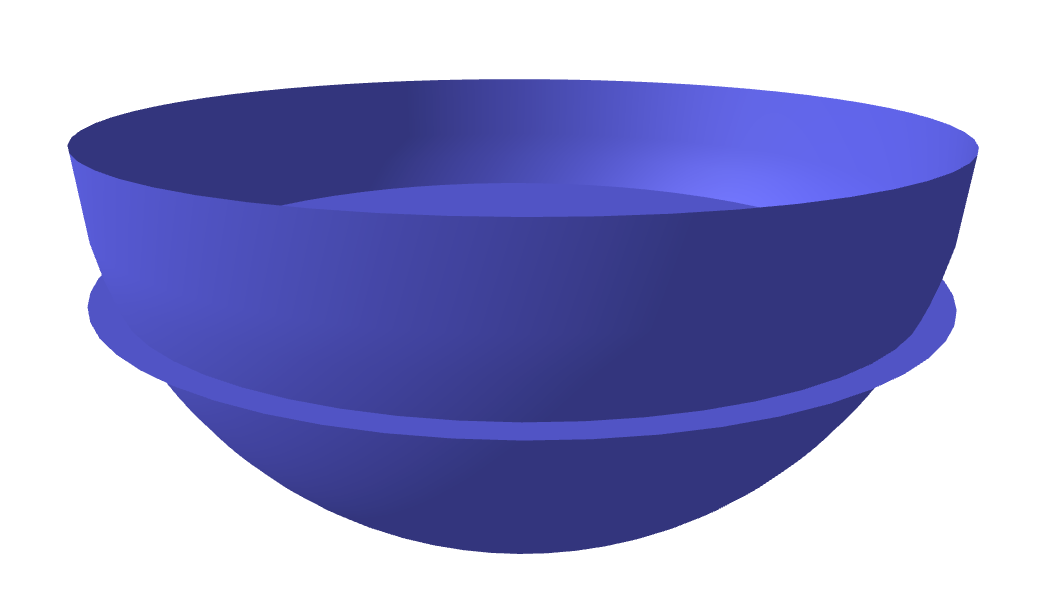You never know what math problems will show up in applications. I once had a passing thought to include the following problem on a calculus test, but I sure as heck am glad I didn't (I have included variations which seemed just slightly different from this, but solving for a different variable). Anyway so here's the story. I use measuring spoons (and cups) when I cook (I may already cause offense by doing so, lol). Notably, my measuring spoons are hemispherical. I needed a half tablespoon of something. A bit of soy sauce, being the Real Asian™ that I am. I'm missing a few teaspoon measures, due to a mishap in the garbage disposer (oops). So not having my usual measures for 1½ teaspoons (or 3/2 teaspoons, since mathematicians like improper fractions), involving very easy math, like multiplication by 3, I decided, well, why don't I just fill the hemisphere to the exact height needed to get a volume of half a hemisphere? Easy peasy. Shouldn't be much harder than multiplying by 3, right? Well... turns out to involve the number 3 in something way, way more complicated.

Now the calculus problem that I have definitely put on exams is: if you fill a hemisphere (of radius $R$) to exactly half the height ($\frac{1}{2} R$), what's the volume of such a thing? Now of course, because the curve of the hemisphere changes with the height, filling to the halfway height point is going to give you less than half the volume. Realizing the volume as a stack of disks, the answer is

$\int_{R/2}^{R} \pi(R^2 - z^2) \; dz = \frac{2\pi}{3} R^3 - \int_0^{R/2} \pi(R^2 - z^2) \; dz = \frac{2\pi}{3} R^3 - \pi R^2 z + \frac{\pi}{3} z^3 = \frac{2\pi}{3}R^3 - \frac{\pi}{2} R^3 +\frac{\pi}{3\cdot 8} R^3 = \frac{5\pi}{24} R^3.$

Oh. I thought it involved $\sqrt{2} - 1$ or something. Oh well. My memory is not what it used to be, I guess. I think that may be the answer to my volume-of-a-Sno-Cone problem, lol, which is the volume of the segment of spherical cap cut off by a cone. I don't feel like busting out the spherical coordinates today, anyway, to do that problem, lol. Some other time!

Anyway, this is of course, not half a hemisphere's worth, which is $\frac{1}{3} \pi R^3$. To get that, we have to solve for the height. It is easier to measure from the top of the spoon ($xy$-plane) than from the bottom, so we solve

$\frac13 R^3 = \int_0^h \pi(R^2 - z^2) \; dz = hR^3-\frac{1}{3} \pi h^3.$

for $h$. Simple, right? Clearing denominators and moving stuff to the other side, and canceling off the pesky $\pi$, we end up with

$h^3 - 3R^3 h + R^3 = 0$.

A nontrivial cubic polynomial. As it happens, this is the case of the depressed cubic (insert many jokes/puns here and catch flack from the sensitivity police for making light of serious, real mental illnesses). Of course, using Mathematician's Privilege (dimensional consistency is such a ... physicist ... thing), we can just set our units to $1$ and be done with it; this gives us the polynomial

$h^3 -3h + 1 = 0$.

Now I could have also tried to have my college algebra students solve this one. Rational roots theorem and all that. Nope, not gonna work, throwing $1$ and $-1$ at it. It turns out that this one is a true casus irreducibilis. It being a depressed cubic, I don't have to deal with the Germans, and can skip directly to Italians with fancy cars (perhaps I'll "retcon" my story and say I was cooking a nice risotto and needed to use half a hemispherical teacup), the solution to this is

$\sqrt{-\frac{1}{2} + \sqrt{\frac{1}{4} - 1}} + \sqrt{-\frac{1}{2} - \sqrt{\frac{1}{4} - 1}}$,

$\zeta\sqrt{-\frac{1}{2} + \sqrt{\frac{1}{4} - 1}} + \bar\zeta\sqrt{-\frac{1}{2} - \sqrt{\frac{1}{4} - 1}}$,

$\bar \zeta\sqrt{-\frac{1}{2} + \sqrt{\frac{1}{4} - 1}} + \zeta \sqrt{-\frac{1}{2} -\sqrt{\frac{1}{4} - 1}}$, where $\zeta$ is a cube root of unity (please print these formulas out onto posters and hold them up to enforce social distancing). It happens that $\zeta = -\frac{1}{2} + \frac{\sqrt{3}}{2}\mathsf i$, which is exactly what is under the cube root, so we're dealing with ninth roots of unity here! (who would have thought??). The way to see that these formulas work, generally from the depressed cubic, is basically to substitute $x = w - \frac{p}{3w}$, which results in a quadratic for $w^3$. That it's quadratic explains the nearly identical features of the stuff under the cube roots, but for a sign, and that it's $w^3$ that gets solved for is responsible for the cube roots outside, as well as mutiplying by roots of unity.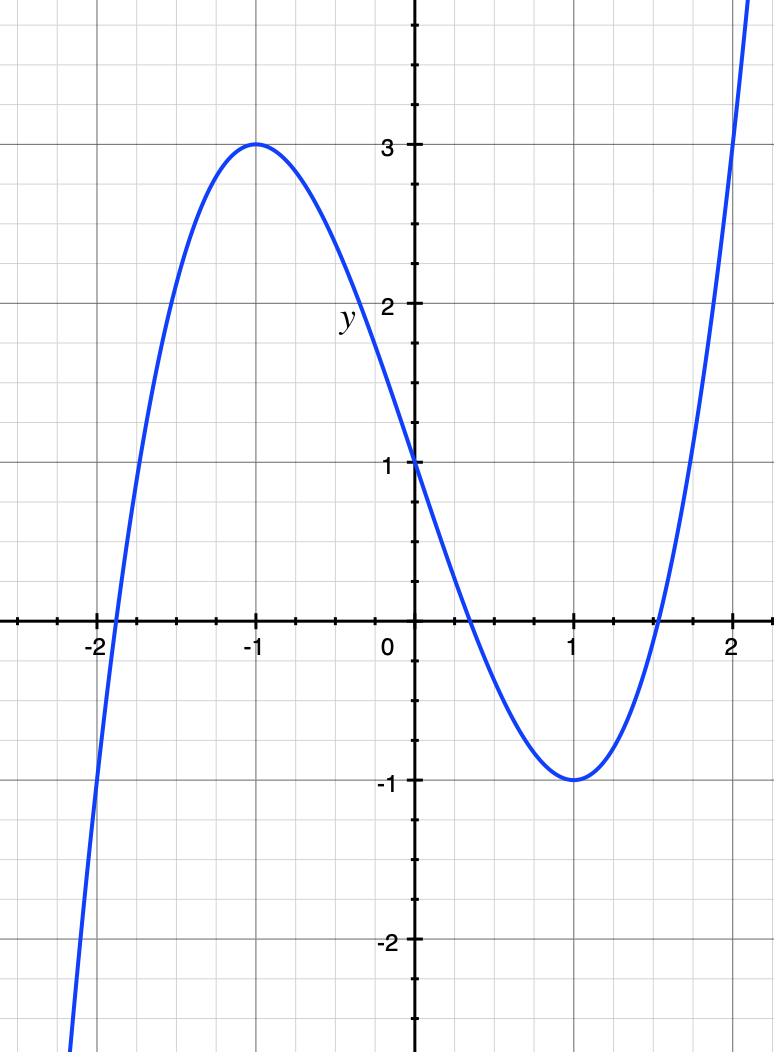The cubic $y = x^3 - 3x + 1$. It has three real roots, but they only can be expressed algebraically by the sum of complex numbers!

So the three solutions are $\sqrt{\zeta} + \sqrt{\bar{\zeta}} = \zeta^{1/3} + \zeta^{-1/3}$, $\zeta^{4/3} + \zeta^{-4/3}$, and $\zeta^{-2/3} + \zeta^{2/3}$. Taking the cube root of a complex number is not a trivial matter. It happens that the imaginary parts all cancel out and you get three real solutions, but this cannot be done without admitting complex numbers in some form (after now consulting with the French). Well, almost, anyway. Let's call in trigonometry to save the day (but that's cheating, because trigonometry really is $e^{\mathsf i x}$). We'll take $\zeta = e^{2\pi\mathsf i/3}$ and the "principal" complex cube root to take minimum argument. Actually this is irrelevant, because the extra multiplications of the $\zeta$s give you the other cube roots anyway, but for definiteness... $\zeta^{1/3}$ then is $e^{2\pi\mathsf i / 9}$ and the possible solutions are

$e^{2\pi\mathsf i / 9} + e^{-2\pi\mathsf i / 9} = 2\cos(2\pi/9)$
$e^{8\pi\mathsf i / 9} + e^{-8\pi\mathsf i / 9} = 2\cos(8\pi/9)$, and
$e^{-4\pi\mathsf i / 9} + e^{4\pi\mathsf i / 9} = 2\cos(4\pi/9)$.

Ok, so which one of these solutions is the correct one? The solution has to be between $-1$ and $1$ (in our units; the original units would be between $-R$ and $R$), and as such, only $2 \cos(4\pi/9) \approx 0.3472$ (the middle one in the graph of the full cubic above) works. So to answer the question, you need to fill up the tablespoon measure so that it is 34.72% of the way down from the top. Here's another picture and zoomed in: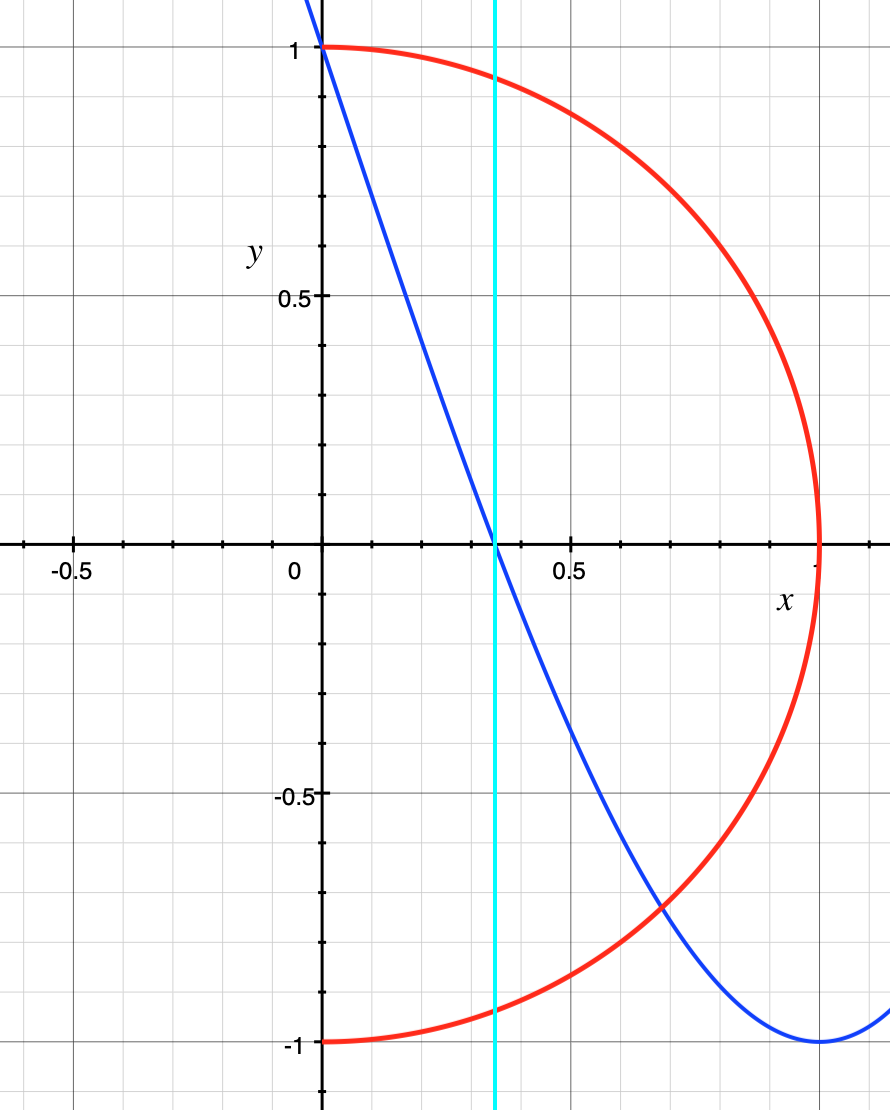A cross section of the hemisphere in question, together with a zoomed in view of the cubic curve (green). The vertical line (cyan) drawn through the cubic at its root will intersect the circle (red) where it needs to be filled (here you'd fill everything to the right of this line)

Again the fact that solving a cubic has to go through the complex numbers is why complex numbers were finally accepted. It is not, as commonly believed, due to trying to solve for the quadratic, since in that case, complex numbers only appear when the parabola fails to intersect the $x$-axis, meaning the complex solutions are of the impossible, nonexistent intersection points, so, the various Renaissance mathematicians thought, obviously should not be taken seriously. But the cubic forces it to happen no matter what, because a cubic curve always crosses the $x$-axis. So there it is: half a tablespoon? You'll need complex numbers for that. Some more views (the title image sort of is looking from above, so might obscure the fact you must fill it more than halfway from the bottom):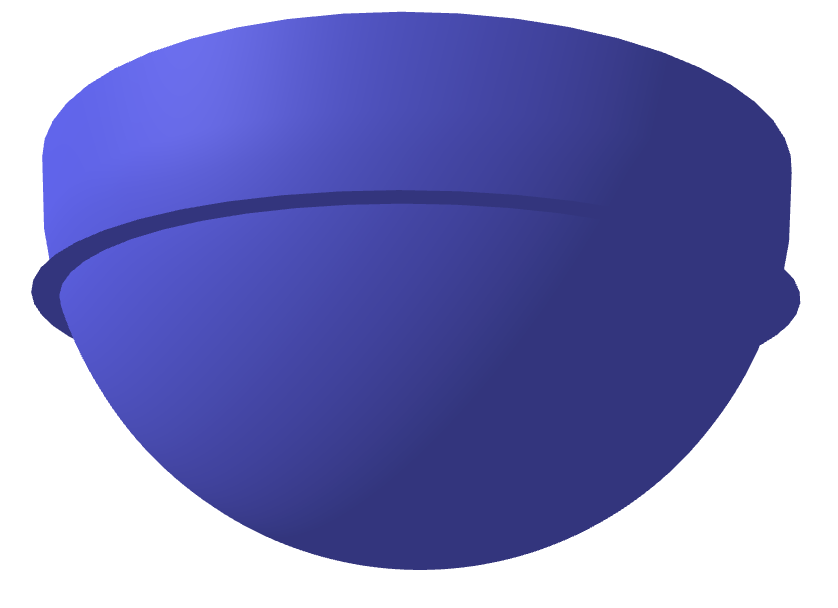View from below the tablespoon. The little extra disk sticking out is the fill line.

Finally, I'll end with more social distancing formulas: the full cubic formula $ax^3 + bx^2 + cx + d = 0$: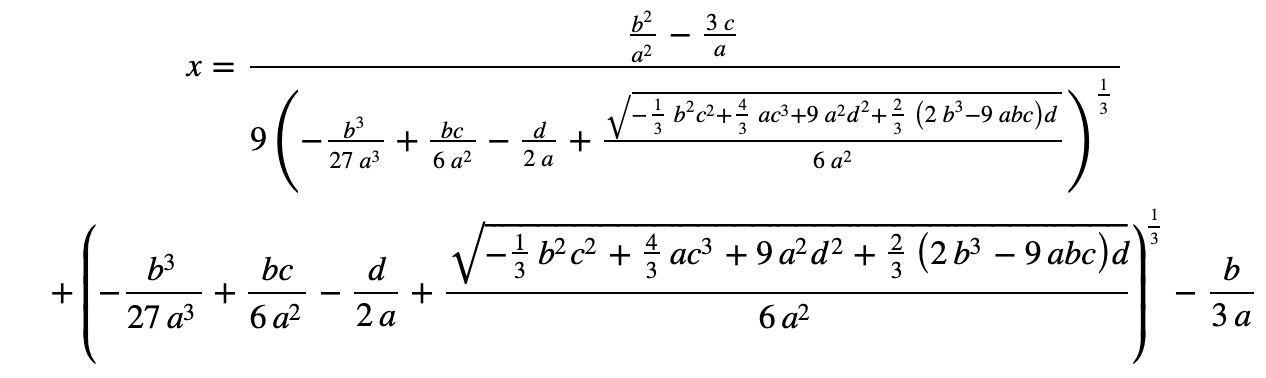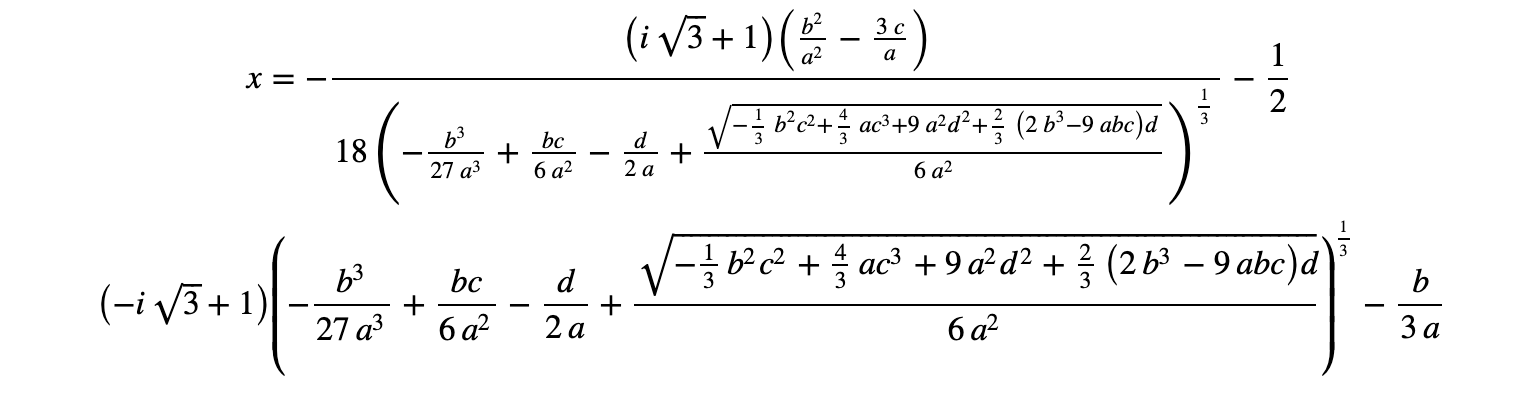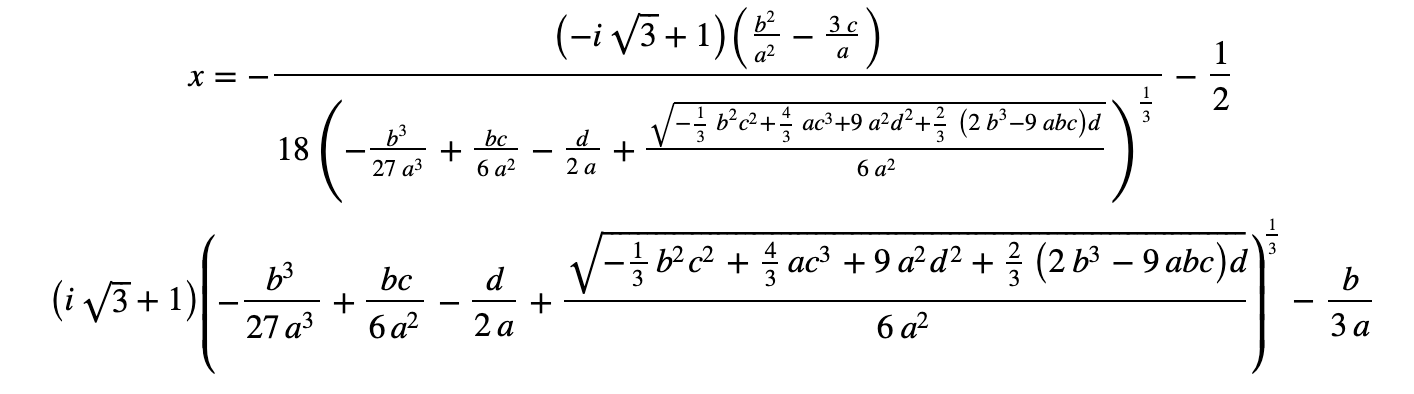For those who are interested, here's a nice video explaining this formula, and why we don't teach it in high school...

It's not taught because the solutions you get may not be easily reducible to something you can recognize, even in simple cases. Conceptually, however, it is a real adventure, and solving it by breaking it into a 2-step process makes it tractable and interesting. Our tablespoon example already skips step 1 ... all that about Germans and Italians.

Enjoy and stay safe!